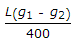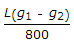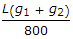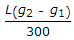# Civil Engineering - Highway Engineering

### Exercise :: Highway Engineering - Section 3

26.

Curves in the same direction separated by short tangents, are called

 A. simple circular curves B. compound curves C. transition curves D. broken-back curves. E. None of these.

Explanation:

No answer description available for this question. Let us discuss.

27.

When an up gradient of a highway meets a downgrade, the vertical curve provided, is known as

 A. valley curve B. sag curve C. summit curve D. all the above.

Explanation:

No answer description available for this question. Let us discuss.

28.

If the coefficient of friction on the road surface is 0.15 and a maximum super-elevation 1 in 15 is provided, the maximum speed of the vehicles on a curve of 100 metre radius, is

 A. 32.44 km/hour B. 42.44 kg/hour C. 52.44 km/hour D. 62.44 km/hour E. 72.44 km/hour

Explanation:

No answer description available for this question. Let us discuss.

29.

The maximum distance of the apex of a vertical curve of length L from the point of intersection of two grades + g1%, and - g2% (g1 > g2), is

 A.B.C.D.Explanation:

No answer description available for this question. Let us discuss.

30.

The wall constructed for the stability of a back filling portion of a road on the down hill side, is known as

 A. retaining wall B. breast wall C. parapet wall D. all the above.